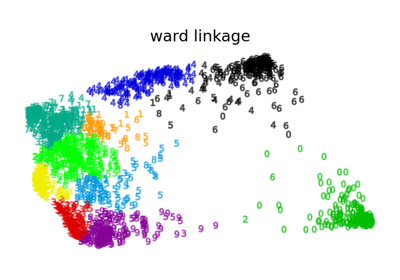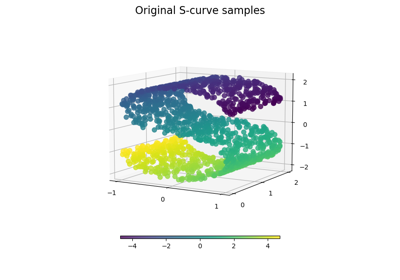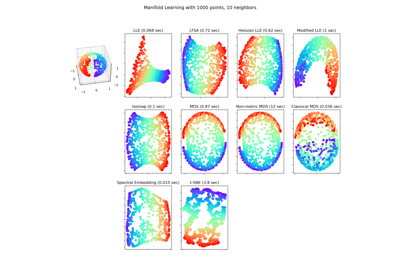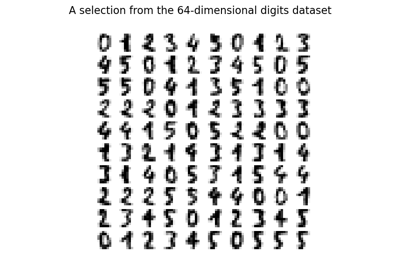# sklearn.manifold.SpectralEmbedding¶

class sklearn.manifold.SpectralEmbedding(n_components=2, *, affinity='nearest_neighbors', gamma=None, random_state=None, eigen_solver=None, n_neighbors=None, n_jobs=None)[source]

Spectral embedding for non-linear dimensionality reduction.

Forms an affinity matrix given by the specified function and applies spectral decomposition to the corresponding graph laplacian. The resulting transformation is given by the value of the eigenvectors for each data point.

Note : Laplacian Eigenmaps is the actual algorithm implemented here.

Read more in the User Guide.

Parameters
n_componentsint, default=2

The dimension of the projected subspace.

affinity{‘nearest_neighbors’, ‘rbf’, ‘precomputed’, ‘precomputed_nearest_neighbors’} or callable, default=’nearest_neighbors’
How to construct the affinity matrix.
• ‘nearest_neighbors’ : construct the affinity matrix by computing a graph of nearest neighbors.

• ‘rbf’ : construct the affinity matrix by computing a radial basis function (RBF) kernel.

• ‘precomputed’ : interpret X as a precomputed affinity matrix.

• ‘precomputed_nearest_neighbors’ : interpret X as a sparse graph of precomputed nearest neighbors, and constructs the affinity matrix by selecting the n_neighbors nearest neighbors.

• callable : use passed in function as affinity the function takes in data matrix (n_samples, n_features) and return affinity matrix (n_samples, n_samples).

gammafloat, default=None

Kernel coefficient for rbf kernel. If None, gamma will be set to 1/n_features.

random_stateint, RandomState instance or None, default=None

Determines the random number generator used for the initialization of the lobpcg eigenvectors when solver == ‘amg’. Pass an int for reproducible results across multiple function calls. See :term: Glossary <random_state>.

eigen_solver{‘arpack’, ‘lobpcg’, ‘amg’}, default=None

The eigenvalue decomposition strategy to use. AMG requires pyamg to be installed. It can be faster on very large, sparse problems. If None, then 'arpack' is used.

n_neighborsint, default=None

Number of nearest neighbors for nearest_neighbors graph building. If None, n_neighbors will be set to max(n_samples/10, 1).

n_jobsint, default=None

The number of parallel jobs to run. None means 1 unless in a joblib.parallel_backend context. -1 means using all processors. See Glossary for more details.

Attributes
embedding_ndarray of shape (n_samples, n_components)

Spectral embedding of the training matrix.

affinity_matrix_ndarray of shape (n_samples, n_samples)

Affinity_matrix constructed from samples or precomputed.

n_neighbors_int

Number of nearest neighbors effectively used.

References

Examples

>>> from sklearn.datasets import load_digits
>>> from sklearn.manifold import SpectralEmbedding
>>> X, _ = load_digits(return_X_y=True)
>>> X.shape
(1797, 64)
>>> embedding = SpectralEmbedding(n_components=2)
>>> X_transformed = embedding.fit_transform(X[:100])
>>> X_transformed.shape
(100, 2)


Methods

 fit(X[, y]) Fit the model from data in X. fit_transform(X[, y]) Fit the model from data in X and transform X. get_params([deep]) Get parameters for this estimator. set_params(**params) Set the parameters of this estimator.
fit(X, y=None)[source]

Fit the model from data in X.

Parameters
X{array-like, sparse matrix} of shape (n_samples, n_features)

Training vector, where n_samples is the number of samples and n_features is the number of features.

If affinity is “precomputed” X : {array-like, sparse matrix}, shape (n_samples, n_samples), Interpret X as precomputed adjacency graph computed from samples.

yIgnored
Returns
selfobject

Returns the instance itself.

fit_transform(X, y=None)[source]

Fit the model from data in X and transform X.

Parameters
X{array-like, sparse matrix} of shape (n_samples, n_features)

Training vector, where n_samples is the number of samples and n_features is the number of features.

If affinity is “precomputed” X : {array-like, sparse matrix} of shape (n_samples, n_samples), Interpret X as precomputed adjacency graph computed from samples.

yIgnored
Returns
X_newarray-like of shape (n_samples, n_components)
get_params(deep=True)[source]

Get parameters for this estimator.

Parameters
deepbool, default=True

If True, will return the parameters for this estimator and contained subobjects that are estimators.

Returns
paramsdict

Parameter names mapped to their values.

set_params(**params)[source]

Set the parameters of this estimator.

The method works on simple estimators as well as on nested objects (such as Pipeline). The latter have parameters of the form <component>__<parameter> so that it’s possible to update each component of a nested object.

Parameters
**paramsdict

Estimator parameters.

Returns
selfestimator instance

Estimator instance.

## Examples using sklearn.manifold.SpectralEmbedding¶Various Agglomerative Clustering on a 2D embedding of digits¶Comparison of Manifold Learning methods¶Manifold Learning methods on a severed sphere¶Manifold learning on handwritten digits: Locally Linear Embedding, Isomap…¶